GeeksforGeeks App
Open AppBrowser
Continue

# Class 10 NCERT Solutions- Chapter 12 Areas Related to Circles – Exercise 12.3 | Set 1

### Question 1. Find the area of the shaded region in Fig., if PQ = 24 cm, PR = 7 cm and O is the center of the circle.

Solution:

In fig. By Pythagoras theorem

RQ2=RP2+PQ2

RQ2=(7)2+(24)2

RQ2=625

RQ=√625

RQ=√5*5*5*5

RQ=5*5

=25

Areas of shaded region=Area of semi circle -Area of ∆RPQ

=1/2πR2-1/2*b*h

=(1/2*22/7*25/2)-(1/2*7*24)

=-84

=161.53cm2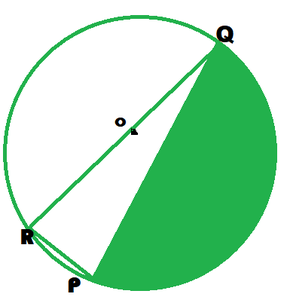### Question 2. Find the area of the shaded region in Fig., if radii of the two concentric circles with center O are 7 cm and 14 cm respectively and ∠AOC = 40°.

Solution:

Area of shaded Region=Area of sector AOC – Area of sector BOD

∠AOC = θ

Radius of inner circle = r

Radius of outer circle = R

=θ/360 πR2 – θ/360 πr2

= θ/360 π(R2-r2)

=40/(360)*22/7(14*14-7*7)

=40/360*22/7(196-49)

=(22/63) * 147

=154/3

=cm2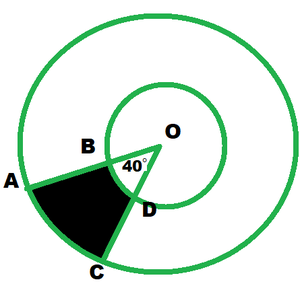### Question 3. Find the area of the shaded region in Fig., if ABCD is a square of side 14 cm and APD and BPC are semicircles.

Solution:

Area of shaded region=Area of square-Area of 2 semi-circles

=side*side-2*1/2πr2

=14*14 – 22/7*7*7

=196-154

=42 cm2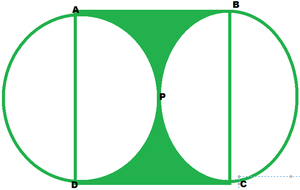### Question 4. Find the area of the shaded region in Fig., where a circular arc of radius 6 cm has been drawn with vertex O of an equilateral triangle OAB of side 12 cm as center.

Solution:

Area of shaded region=Area of big sector+ Area of equilateral

=θ/360 πr2+√3/4(side)2

=(300/360)*22/7*6*6+(√3/4)*12*12

=5/6*22/7*6*6+36*1.73

=660/7+62.28

=94.28+62.28

=156.56 cm2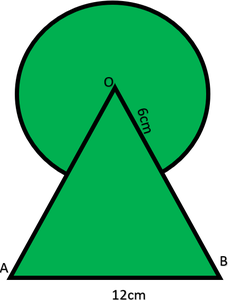### Question 5. From each corner of a square of side 4 cm a quadrant of a circle of radius 1 cm is cut and also a circle of diameter 2 cm is cut as shown in Figure. Find the area of the remaining portion of the square.

Solution:

Area of remaining poison=Area of square – Area of 4 quadrants – Area of circle in the middle

=side*side – 4(90/360 πr2) – πr2

=4*4 – 2 πr2

=16-2*22/7*1*1

=16-44/7

=9.72cm2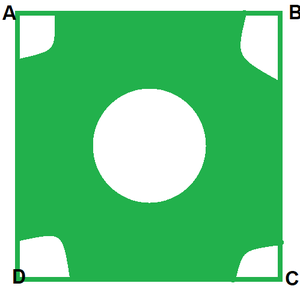### Question 6. In a circular table cover of radius 32 cm, a design is formed leaving an equilateral triangle ABC in the middle as shown in Figure. Find the area of the design.

Solution:

Area of circle= πr2=22/7*32*32=22528/7=3218.28cm2     —–1

Area of ∆ABC=3*Area of ∆BOC

=3*1/2*side*side*sin120°

=3/2*32*32*sin60°

=1536*√3/2

=768*1.73

=1328.64cm2     ———2

Area of design =Area of circle – Area of ∆ABC

=321.28-1328.64

=1889.64 cm2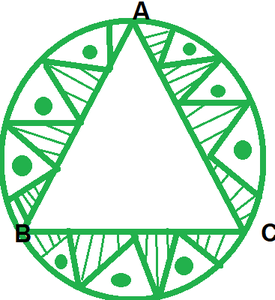### Question 7.In Fig., ABCD is a square of side 14 cm. With centers A, B, C and D, four circles are drawn such that each circle touch externally two of the remaining three circles. Find the area of the shaded region.

Solution:

Area of shaded region=Area of square – Area of 4 quarters

=side*side – 4(90/360 πr2)

=14*14-22/7*7*7

=196-154

=42cm2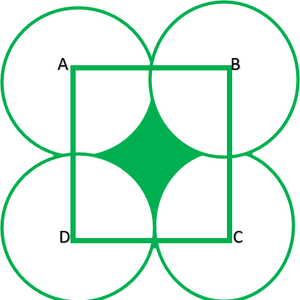### Question 8. Fig. here depicts a racing track whose left and right ends are semi-circular. The distance between the two inner parallel line segments is 60 m and they are each 106 m long. If the track is 10 m wide, find:

i) the distance around the track along its inner edge

ii) the area of the track

Solution:

i) A distance along inner edge=length of 2 parallel lines+ circumference of 2 circles

=106+106+2πr

=212+2*22/7*30

=212+188.57

=400.57m

ii) Area of track=Area of 2 rectangles+ semi rings

=106*10*2+π (R-r)2

=2120+22/7((40)2-(30)2)

=210+22/7(1600-900)

=210+2200

=4320 m2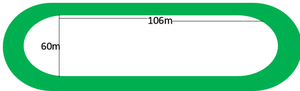### Question 9. In Fig., AB and CD are two diameters of a circle (with center O) perpendicular to each other and OD is the diameter of the smaller circle. If OA = 7 cm, find the area of the shaded region.

Solution:

Area of smaller circle=πr2

=22/7*7/2*7/2

=78/2 =38.5m2

Area of segment=Area of quadrant-Area of ∆BOC

=1/4πR2-1/2*BO*OC

=1/4*22/7*7*7-1/2*7*7

=77/2-49/2

=77-49/2

=28/2

=14 cm2

Area of shaded region=Area of smaller circle+2*Area of segment

=38.5 + 2*14

=38.5+28

=66.5 cm2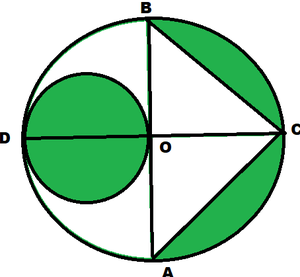### Question 10. The area of an equilateral triangle ABC is 17320.5 cm2. With each vertex of the triangle as center, a circle is drawn with radius equal to half the length of the side of the triangle (see Fig.). Find the area of the shaded region. (Use π = 3.14 and √3 = 1.73205)

Solution:

Area of equilateral ∆ =1.73205

π=3.14

√3=1.73205

√3/4 (side)2=173205

1.73205/4*(side)2=1.73205

(side)2=173205*4/1.73205

(side)2=173205*100000/10*173205

=√1000*4

=√(100 *100*2*2)

=100*2

=200cm

Area of shaded region=Area of∆ ABC-3*Area of sector

=1.73205*60/360*3.14*100*100

=1.73205-31400/2

=17320.5-15700

=1620.5cm2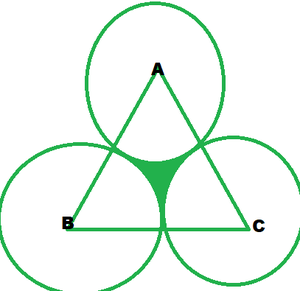### Chapter 12 Areas Related to Circles – Exercise 12.3 | Set 2

My Personal Notes arrow_drop_up
Related Tutorials